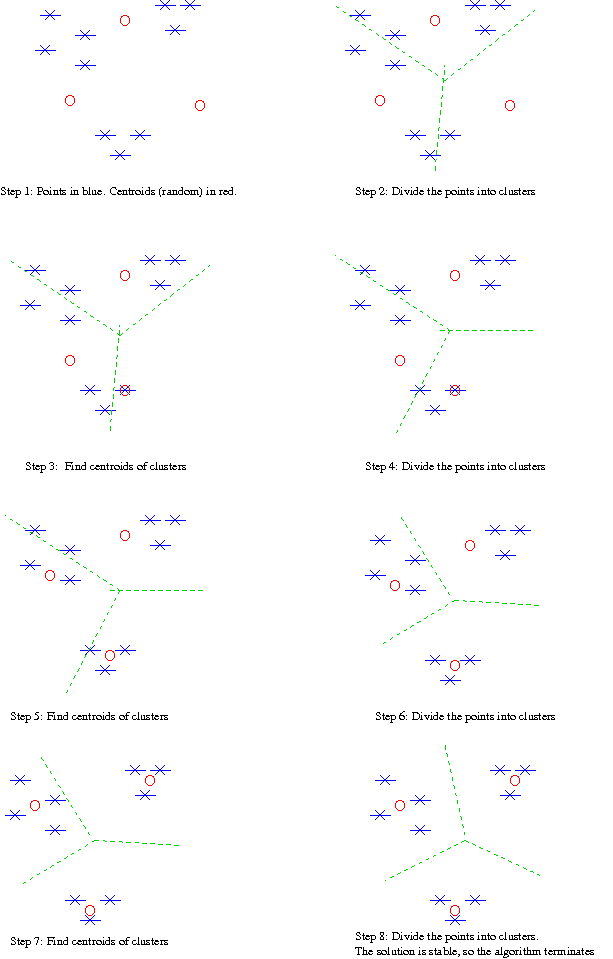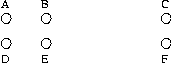## kMeans Clustering

### K-means algorithm

```K-means-cluster (in S : set of vectors : k : integer)
{  let C ... C[k] be a random partition of S into k parts;
repeat {
for i := 1 to k {
X[i] := centroid of C[i];
C[i] := empty
}
for j := 1 to N {
X[q] := the closest to S[j] of X ... X[k]
} }
until the change to C (or the change to X) is small enough.
}

```

Example: (Note: I have started here with random points rather than a random partition.)Features:

• Objective function: minimize the sum of the squared distance from each point to centroid of cluster.
For unit vectors, this is equivalent to maximizing the average dot product S[i] dot S[j} where S[i] and S[j] are in the same cluster.

Sum of the squared distance steadily decreases over the iterations.
Hill-climbing algorithm.

• Disjoint and exhaustive decomposition.
• For fixed number of iterations, linear in N. Clever optimization reduces recomputation of X[q] if small change to S[j]. Second loop much shorter than O(kN) after the first couple of iterations.
• "Anytime" algorithm: S[j] always a decomposition of S into convex subregions.
• Random starting point: Multiple runs may give different results, choose "best"

Problems:

• Have to guess K.
• Local minimum. Example: In diagram below, if K=2, and you start with centroids B and E, converges on the two clusters {A,B,C}, {D,E,F}• Disjoint and exhaustive decomposition.
• Starvation: Complete starvation of C[j], or starvation to single outlier.
• Assumes that clusters are spherical in vector space. Hence particularly sensitive to coordinate changes (e.g. changes in weighting)
• Worst-case running time is exponential in pathological cases: 2cn for some constant c>0 (see k-means Requires Exponentially Many Iterations Even in the Plane Andrea Vattani, 2011,) However, (a) in practice it generally converges quickly; (b) if there are dubious points near the boundary between, then it's not very important to get the assignment exactly right.

Variant 1: Update centroids incrementally. Claims to give better results.

Variant 2: Bisecting K-means algorithm:

```for I := 1 to K-1 do {
pick a leaf cluster C to split;
for (J := 1 to ITER)
use 2-Means clustering to split C into two subclusters C1 and C2;
choose the best of the above splits and make it permanent
}
```
Generates a binary clustering hierarchy. Works better (so claimed) than K-means.

Variant 3: Allow overlapping clusters: If distances from P to C1 and to C2 are close enough then put P in both clusters.

### Efficient implementation of K-means clustering

As we wrote the algorithm before, on each iteration you have to compute the distance from every point to every centroid. If there are many points, and K is reasonably large, and the division into clusters has become fairly stable, then the update procedure can be made more efficient as follows:

First, the computation of the new centroid requires only the points added to the cluster, the points taken out of the cluster, and the number of points in the cluster.

Second, computing the change made to the cluster made by moving the centroid can be made more efficient as follows:

Let XJ(T) be the centroid of the Jth cluster on the Tth iteration, and let VI be the Ith point. Note that
dist(VI,XJ(T+1)) <= dist(VI,XJ(T)) + dist(XJ(T),XJ(T+1).)

Therefore you can maintain two arrays:

RADIUS(J) = an overestimate of the maximum value of dist(VI,XJ), where VI is in cluster J.

DIST(J,Z) = an underestimate of the minimum value of dist(VI,XZ), where VI is in cluster J.

which you update as follows:

DIST(J,Z) := DIST(J,Z) - dist(XZ(T),XZ(T+1)) - dist(XJ(T),XJ(T+1));
then as long as RADIUS(J) < DIST(J,Z) you can be sure that none of the points in cluster J should be moved to cluster Z. (You update these values more exactly whenever a point is moved.)

#### Sparse centroids

The centroid of a collection of documents contains a non-zero component corresponding to every term that appears in any of the documents. Therefore it is a much less sparse vector than the individual documents, which can incur computational costs. One solution is to limit the number of non-zero terms, or threshhold them. Another is to replace the centroid of a set C by the ``most central'' point in U. There are a number of ways to define this; for instance choose the point X that minimizes maxV in C d(X,V) or that minimizes sumV in C d(X,V)2

This can be approximated in linear time as follows:

```medoid(C,X) {
U := the point in C furthest from X;
V :- the point in C furthest from U;
Y := the point in C that minimizes d(Y,U) + d(Y,V) + |d(Y,U)-d(Y,V)|
return(Y)
}
```
Note that d(X,U)+d(X,V) is minimal, and equal to d(U,V) for points X on the line between U and V, and for points on that line |d(X,U)-d(X,V)| is minimal when X is at the center of the line. [Geraci et al. 2006] call this the "medoid" of C.

#### Finding the right value of K

Let S be a system of clusters.
Let S[d] be the cluster containing document d.
Let m(C) be the median of cluster C.
For a system of clusters S, let RSS(S) = Σd (d-m(S(d)))2

For K=1,2, ... compute SK = best system with K clusters.
Plot RSS(SK) vs. K. Look for a "knee" in plot. Choose that as value of K.
Question to which I don't know the answer: Is this curve always concave upward?